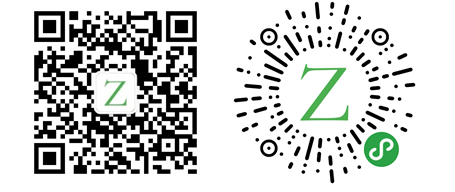# 走进 Rust：智能指针

Rust 大约 1085 字

## 标准库中的智能指针

• `String`：字符串
• `Vector<T>`：集合
• `Box<T>`：用于在堆上分配值
• `Rc<T>`：一个引用计数类型，其数据可以有多个所有者
• `Ref<T>``RefMut<T>`：通过`efCell<T>`访问。（`RefCell<T>`是一个在运行时而不是在编译时执行借用规则的类型）

## 元祖结构体

``````struct Color(i32, i32, i32);
struct Point(i32, i32);
struct Test(u8);

fn main() {
let c = Color(0, 255, 100);
let p = Point(10, 20);
let t = Test(10);
}``````

`Box<T>`被定义为包含一个元素的元组结构体。

``````pub struct Box<
T: ?Sized,
#[unstable(feature = "allocator_api", issue = "32838")] A: Allocator = Global,
>(Unique<T>, A);``````

``````struct MyBox<T>(T);

impl<T> MyBox<T> {
fn new(x: T) -> MyBox<T> {
MyBox(x)
}
}

impl<T> Deref for MyBox<T> {
type Target = T;

fn deref(&self) -> &T {
&self.0
}
}``````

————        END        ————

Give me a Star, Thanks:)

https://github.com/fendoudebb